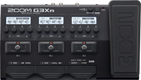# Blues tone

Discussion in 'Zoom G3n/G3Xn/G5n' started by Peter Lucas, Oct 14, 2021.

1. Blues toneDevice: Zoom G3Xn
Firmware: 2.20

Name on device: ECSTRATBLU
Optimized for: Guitar Amp

Effects chain:Sort of Eric Clapton tone?

Effect: "ZNR" (Dynamics), active - "yes"
"Detect" = GTRIN
"Depth" = 100
"Threshold" = 25
"Decay" = 10

Effect: "HG THRTTL" (Overdrive / Distortion), active - "yes"
"Gain" = 20
"Tone" = 12
"MdCut" = 60
"Volume" = 100

Effect: "UK 30A" (Amp simulator), active - "yes"
"Bass" = 20
"Treble" = 75
"Cut" = 20
"Gain" = 20
"Volume" = 100
"Depth" = 0
"Speed" = 40
"SOLO" = 5

Effect: "BGN4x12" (Cabinet), active - "yes"
"MIC" = ON
"D57: D421" = 50
"Hi" = 60
"Lo" = 35

Effect: "ZNR" (Dynamics), active - "yes"
"Detect" = GTRIN
"Depth" = 100
"Threshold" = 25
"Decay" = 0

Effect: "PDL Vol" (Pedal), active - "yes"
"Volume" = 100
"Min" = 60
"Max" = 100
"Curve" = A

Patch Volume: 95